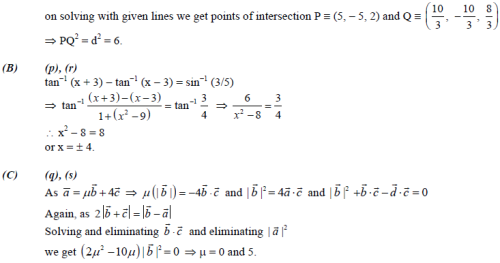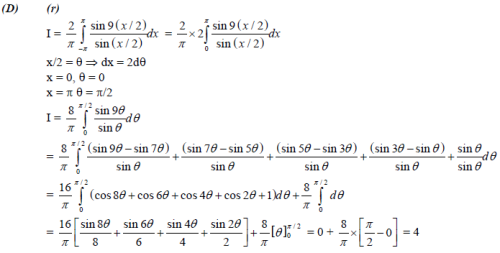Courses

# JEE Advanced 2010 Paper - 2 with Solutions

## 57 Questions MCQ Test Mock Test Series for JEE Main & Advanced 2021 | JEE Advanced 2010 Paper - 2 with Solutions

Description
This mock test of JEE Advanced 2010 Paper - 2 with Solutions for JEE helps you for every JEE entrance exam. This contains 57 Multiple Choice Questions for JEE JEE Advanced 2010 Paper - 2 with Solutions (mcq) to study with solutions a complete question bank. The solved questions answers in this JEE Advanced 2010 Paper - 2 with Solutions quiz give you a good mix of easy questions and tough questions. JEE students definitely take this JEE Advanced 2010 Paper - 2 with Solutions exercise for a better result in the exam. You can find other JEE Advanced 2010 Paper - 2 with Solutions extra questions, long questions & short questions for JEE on EduRev as well by searching above.
QUESTION: 1

### SECTION – I (Single Correct Choice Type) This Section contains 6 multiple choice questions. Each question has four choices A), B), C) and D) out of which ONLY ONE is correct. Q. A block of mass 2 kg is free to move along the x-axis. It is at rest and from t = 0 onwards it is subjected to a time-dependent force F(t) in the x direction. The force F(t) varies with t as shown in the figure. The kinetic energy of the block after 4.5 seconds is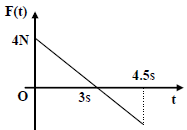Solution: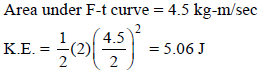QUESTION: 2

Solution:
QUESTION: 3

### A tiny spherical oil drop carrying a net charge q is balanced in still air with a vertical uniform electric field of strength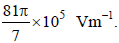When the field is switched off, the drop is observed to fall with terminal velocity 2 × 10−3 ms−1. Given g = 9.8 ms−2, viscosity of the air = 1.8 × 10−5 Ns m−2 and the density of oil = 900 kg m−3, the magnitude of q is

Solution: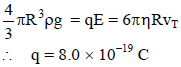QUESTION: 4

A Vernier calipers has 1 mm marks on the main scale. It has 20 equal divisions on the Vernier scale which
match with 16 main scale divisions. For this Vernier calipers, the least count is

Solution: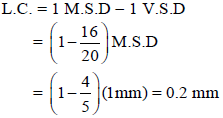QUESTION: 5

A biconvex lens of focal length 15 cm is in front of a plane mirror. The distance between the lens and the mirror is 10 cm. A small object is kept at a distance of 30 cm from the lens. The final image is

Solution:

Object is placed at distance 2 f from the lens. So first image Iwill be formed at distance 2 f on other side. This image I1 will behave like a virtual object for mirror. The second image Iwill be formed at distance 20 cm in front of the mirror, or at distance 10 cm to the left hand side of the lens.

Now applying lens formula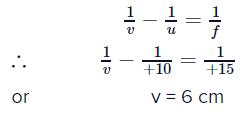Therefore, the final image is at distance 16 cm from the mirror. But, this image will be real.
This is because ray of light is travelling from right to left.
∴ The correct option is (b).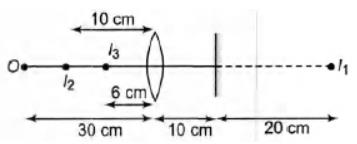QUESTION: 6

A hollow pipe of length 0.8 m is closed at one end. At its open end a 0.5 m long uniform string is vibrating
in its second harmonic and it resonates with the fundamental frequency of the pipe. If the tension in the
wire is 50 N and the speed of sound is 320 ms−1, the mass of the string is

Solution: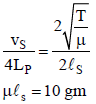QUESTION: 7

SECTION –II (Integer Type)

This Section contains 5 questions. The answer to each question is a single-digit integer, ranging from 0 to 9.

Q. A large glass slab (μ = 5/3) of thickness 8 cm is placed over a point source of light on a plane surface. It is
seen that light emerges out of the top surface of the slab from a circular area of radius R cm. What is the
value of R?

Solution: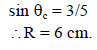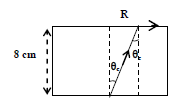*Answer can only contain numeric values
QUESTION: 8

Image of an object approaching a convex mirror of radius of curvature 20 m along its optical axis is
observed to move from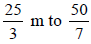m in 30 seconds. What is the speed of the object in km per hour ?

Solution: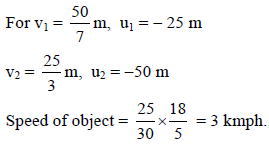QUESTION: 9

To determine the half life of a radioactive element, a student plots a graph of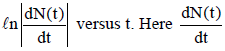is the rate of radioactive decay at time t. If the number of radioactive nuclei of this element decreases by a factor of p after 4.16 years, the value of p is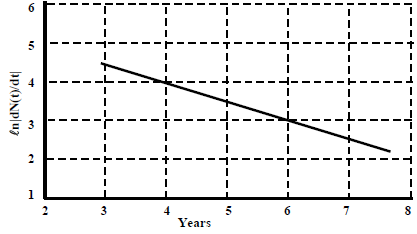Solution: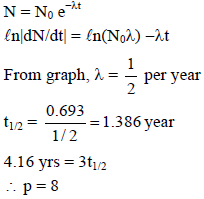*Answer can only contain numeric values
QUESTION: 10

A diatomic ideal gas is compressed adiabatically to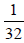of its initial volume. If the initial temperature of
the gas is Ti (in Kelvin) and the final temperature is aTi, the value of a is

Solution: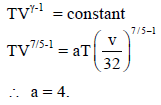*Answer can only contain numeric values
QUESTION: 11

At time t = 0, a battery of 10 V is connected across points A and B in the given circuit. If the capacitors have no charge initially, at what time (in seconds) does the voltage across them becomes 4 volt?

[take ln 5 = 1.6, ln 3 = 1.1]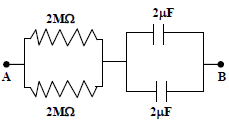Solution: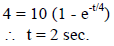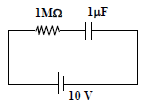QUESTION: 12

SECTION –III (Paragraph Type)

This Section contains 2 paragraphs. Based upon each of the paragraphs 3 multiple choice questions have to be answered. Each of these questions has four choices (A), (B), (C) and (D) out of which ONLY ONE is correct.

Paragraphs for Question 12 To 14

When liquid medicine of density ρ is to be put in the eye, it is done with the help of a dropper. As the bulb on the top of the dropper is pressed, a drop forms at the opening of the dropper. We wish to estimate the size of the drop.

We first assume that the drop formed at the opening is spherical because that requires a minimum increase in its surface energy. To determine the size, we calculate the net vertical force due to the surface tension T when the radius of the drop is R. When the force becomes smaller than the weight of the drop, the drop gets detached from the dropper.

Q. If the radius of the opening of the dropper is r, the vertical force due to the surface tension on the drop of
radius R (assuming r << R) is

Solution: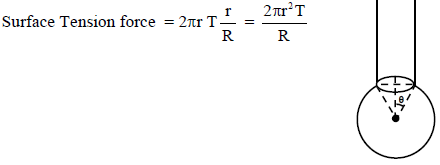QUESTION: 13

Paragraphs for Question 12 To 14

When liquid medicine of density ρ is to be put in the eye, it is done with the help of a dropper. As the bulb on the top of the dropper is pressed, a drop forms at the opening of the dropper. We wish to estimate the size of the drop.

We first assume that the drop formed at the opening is spherical because that requires a minimum increase in its surface energy. To determine the size, we calculate the net vertical force due to the surface tension T when the radius of the drop is R. When the force becomes smaller than the weight of the drop, the drop gets detached from the dropper.

Q. If r = 5 × 10−4 m, ρ = 103 kg m−3, g = 10 m/s2, T = 0.11 Nm−1, the radius of the drop when it detaches from the dropper is approximately

Solution: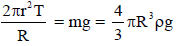QUESTION: 14

Paragraphs for Question 12 To 14

When liquid medicine of density ρ is to be put in the eye, it is done with the help of a dropper. As the bulb on the top of the dropper is pressed, a drop forms at the opening of the dropper. We wish to estimate the size of the drop.

We first assume that the drop formed at the opening is spherical because that requires a minimum increase in its surface energy. To determine the size, we calculate the net vertical force due to the surface tension T when the radius of the drop is R. When the force becomes smaller than the weight of the drop, the drop gets detached from the dropper.

Q. After the drop detaches, its surface energy is

Solution:

Surface energy = T(4πR2) = 2.7 × 10−6 J

QUESTION: 15

Paragraph for questions 15 to 17.

The key feature of Bohr’s theory of spectrum of hydrogen atom is the quantization of angular momentum when an electron is revolving around a proton. We will extend this to a general rotational motion to find quantized rotational energy of a diatomic molecule assuming it to be rigid. The rule to be applied is Bohr’s quantization condition.

Q. A diatomic molecule has moment of inertia I. By Bohr’s quantization condition its rotational energy in the
nth level (n = 0 is not allowed) is

Solution: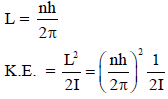QUESTION: 16

Paragraph for questions 15 to 17.

The key feature of Bohr’s theory of spectrum of hydrogen atom is the quantization of angular momentum when an electron is revolving around a proton. We will extend this to a general rotational motion to find quantized rotational energy of a diatomic molecule assuming it to be rigid. The rule to be applied is Bohr’s quantization condition.

Q. It is found that the excitation frequency from ground to the first excited state of rotation for the CO
molecule is close to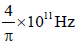. Then the moment of inertia of CO molecule about its centre of mass is
close to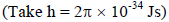Solution: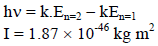QUESTION: 17

Paragraph for questions 15 to 17.

The key feature of Bohr’s theory of spectrum of hydrogen atom is the quantization of angular momentum when an electron is revolving around a proton. We will extend this to a general rotational motion to find quantized rotational energy of a diatomic molecule assuming it to be rigid. The rule to be applied is Bohr’s quantization condition.

Q. In a CO molecule, the distance between C (mass = 12 a.m.u) and O (mass = 16 a.m.u.),
where 1 a.m.u.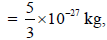is close to

Solution: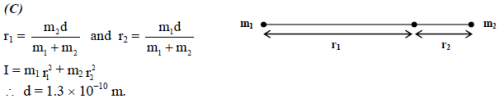QUESTION: 18

SECTION – IV (Matrix Type)

This Section contains 2 questions. Each question has four statements (A, B, C and D) given in Column I and five statements (p, q, r, s and t) in Column II. Any given statement in Column I can have correct matching with one or more statement(s) given in Column II.

Q. Two transparent media of refractive indices μ1 and μ3 have a solid lens shaped transparent material of
refractive index μ2 between them as shown in figures in Column II. A ray traversing these media is also
shown in the figures. In Column I different relationships between μ1, μ2 and μ3 are given. Match them to
the ray diagram shown in Column II.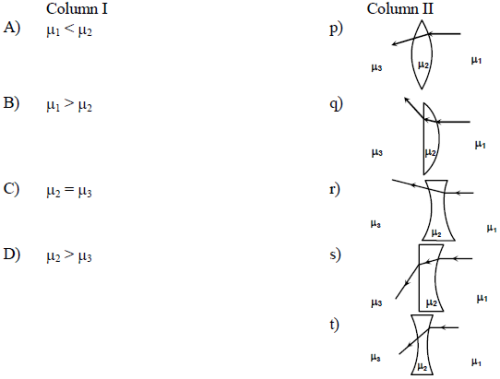Solution:
QUESTION: 19

You are given many resistances, capacitors and inductors. These are connected to a variable DC voltage
source (the first two circuits) or an AC voltage source of 50 Hz frequency (the next three circuits) in
different ways as shown in Column II. When a current I (steady state for DC or rms for AC) flows through
the circuit, the corresponding voltage V1 and V2. (indicated in circuits) are related as shown in Column I.

Match the two

Solution:
QUESTION: 20

SECTION – I (Single Correct Choice Type)

This Section contains 6 multiple choice questions. Each question has four choices A), B), C) and D) out of which ONLY ONE is correct.

Q. The compounds P, Q and S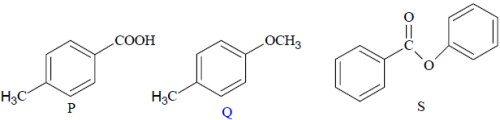were separately subjected to nitration using HNO3/H2SO4 mixture. The major product formed in each case
respectively, is

Solution: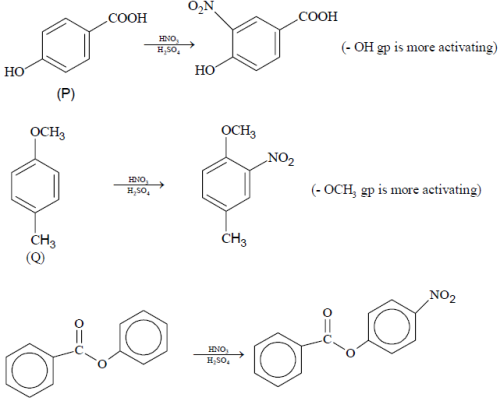QUESTION: 21

Assuming that Hund’s rule is violated, the bond order and magnetic nature of the diatomic molecule B2 is

Solution: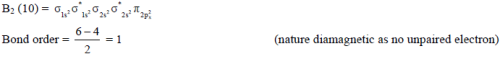QUESTION: 22

The packing efficiency of the two-dimensional square unit cell shown below is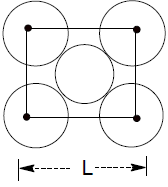Solution: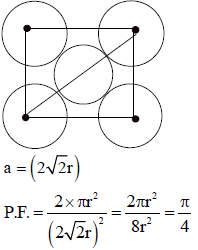QUESTION: 23

The complex showing a spin-only magnetic moment of 2.82 B.M. is

Solution: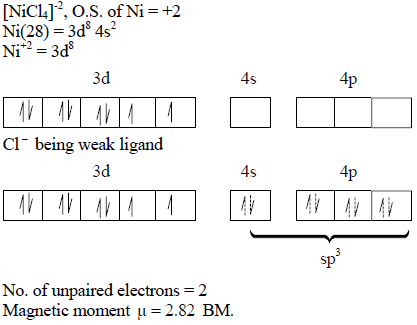QUESTION: 24

In the reaction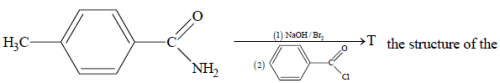Product T is

Solution: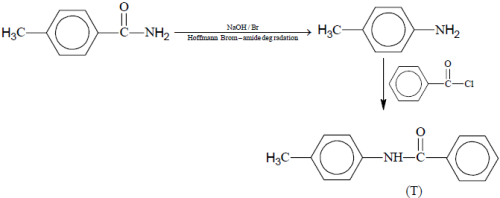QUESTION: 25

The species having pyramidal shape is

Solution: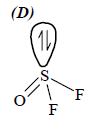*Answer can only contain numeric values
QUESTION: 26

SECTION-II (Integer Type)

This Section contains 5 questions. The answer to each question is a single-digit integer, ranging from 0 to 9.

Enter only the numerical value in the space provided below.

Q. Silver (atomic weight = 108 g mol−1) has a density of 10.5 g cm−3. The number of silver atoms on a surface of area 10−12 m2 can be expressed in scientific notation as y × 10x. The value of x is

Solution: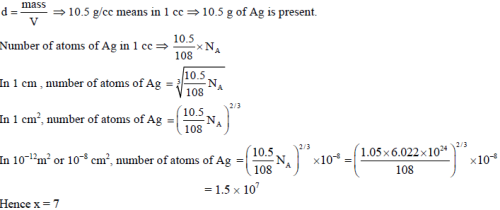*Answer can only contain numeric values
QUESTION: 27

Among the following , the number of elements showing only one non-zero oxidation state is
O, Cl, F, N, P, Sn, Tl, Na, Ti

Solution:

Na, F show only one non-zero oxidation state.

*Answer can only contain numeric values
QUESTION: 28

One mole of an ideal gas is taken from a to b along two paths denoted by the solid and the dashed lines as
shown in the graph below. If the work done along the solid line path is ws and that along the dotted line
path is wd, then the integer closest to the ratio wd/ws is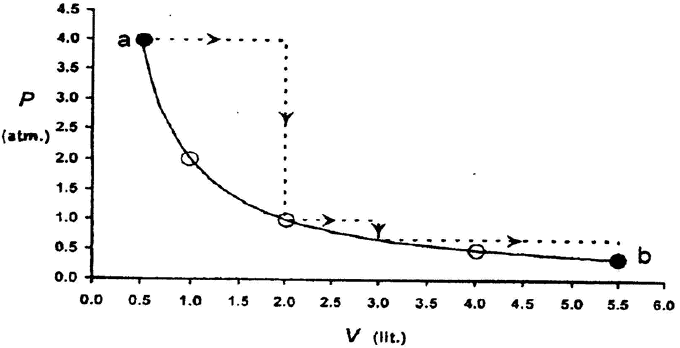Solution: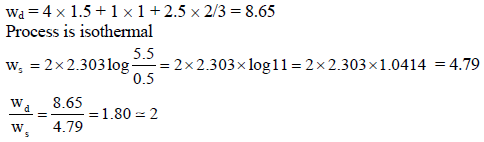*Answer can only contain numeric values
QUESTION: 29

The total number of dirpotic acids among the following is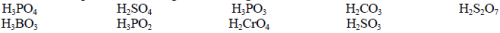Solution:

H2SO4, H2CO3, H2S2O7, H2CrO4, H3PO3, H2SO3

*Answer can only contain numeric values
QUESTION: 30

Total number of geometrical isomers for the complex [RhCl(CO)(PPh3)(NH3)] is

Solution:
QUESTION: 31

SECTION-III (Paragraph Type)

This Section contains 2 paragraphs. Based upon each of the paragraphs 3 multiple choice questions have to be answered. Each of these questions has four choices (A), (B), (C) and (D) out of which ONLY ONE is correct.

Paragraph for questions 31 to 33

Two aliphatic aldehydes P and Q react in the presence of aqueous K2CO3 to give compound R, which upon
treatment with HCN provides compound S. On acidification and heating, S gives the product shown below: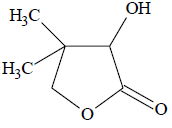Q. The compounds P and Q respectively are

Solution: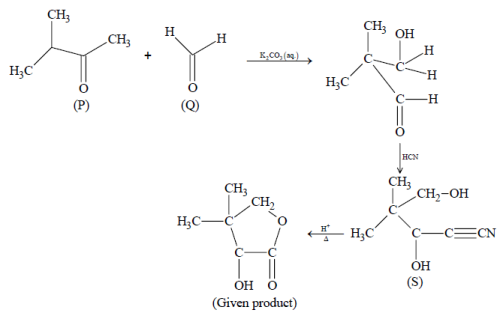QUESTION: 32

Paragraph for questions 31 to 33

Two aliphatic aldehydes P and Q react in the presence of aqueous K2CO3 to give compound R, which upon
treatment with HCN provides compound S. On acidification and heating, S gives the product shown below:The compound R is

Solution:QUESTION: 33

Paragraph for questions 31 to 33

Two aliphatic aldehydes P and Q react in the presence of aqueous K2CO3 to give compound R, which upon
treatment with HCN provides compound S. On acidification and heating, S gives the product shown below:The compound S is

Solution:QUESTION: 34

Paragraph for questions 34 to 36

The hydrogen-like species Li2+ is in a spherically symmetric state S1 with one radial node. Upon absorbing light the ion undergoes transition to a state S2. The state S2 has one radial node and its energy is equal to the ground state energy of the hydrogen atom.

Q. The state S1 is

Solution: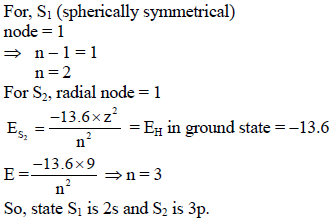QUESTION: 35

Paragraph for questions 34 to 36

The hydrogen-like species Li2+ is in a spherically symmetric state S1 with one radial node. Upon absorbing light the ion undergoes transition to a state S2. The state S2 has one radial node and its energy is equal to the ground state energy of the hydrogen atom.

Q. Energy of the state S1 in units of the hydrogen atom ground state energy is

Solution: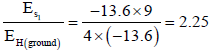QUESTION: 36

Paragraph for questions 34 to 36

The hydrogen-like species Li2+ is in a spherically symmetric state S1 with one radial node. Upon absorbing light the ion undergoes transition to a state S2. The state S2 has one radial node and its energy is equal to the ground state energy of the hydrogen atom.

Q. The orbital angular momentum quantum number of the state S2 is

Solution:

Azimuthal quantum number for S2 = l =1

QUESTION: 37

SECTION-IV (Matrix Type)

This Section contains 2 questions. Each question has four statements (A, B, C and D) given in Column I and five statements (p, q, r, s and t) in Column II. Any given statement in Column I can have correct matching with one or more statement(s) given in Column II.

Q. Match the reactions in Column I with appropriate options in Column II.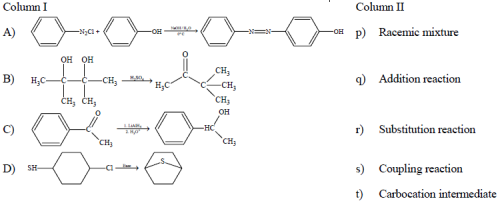Solution:

(A) It is an example of electrophilic substitution reaction which results in coupled product hence it is coupling reaction also.

(B) Pinacole-pinacolone rearrangement. In this reaction intermediate is carbocation.

(C) It is an example of addition reaction by carbonyl compounds and both enantiomers will be formed.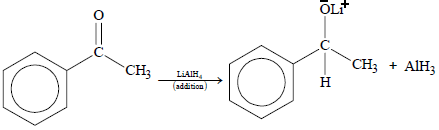Hence, racemic mixture will be obtained.

(D) It is an example of nucleophilic substitution.

QUESTION: 38

All the compounds listed in Column I react with water. Match the result of the respective reactions with the
appropriate options listed in Column II.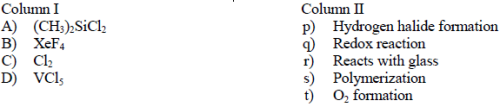Solution: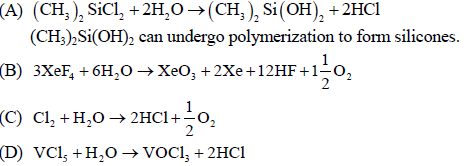QUESTION: 39

SECTION – I (Single Correct Choice Type)

This Section contains 6 multiple choice questions. Each question has four choices A), B), C) and D) out of which ONLY ONE is correct.

Q. If the distance of the point P (1, − 2, 1) from the plane x + 2y − 2z = α, where α > 0, is 5, then the foot of
the perpendicular from P to the plane is

Solution: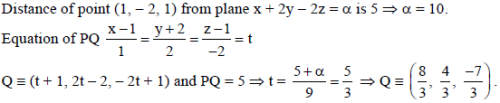QUESTION: 40

A signal which can be green or red with probability 4/5 and 1/5 respectively, is received by station A and then transmitted to station B. The probability of each station receiving the signal correctly is 3/4. If the signal received at station B is green, then the probability that the original signal was green is

Solution:

Event G = original signal is green

E1 = A receives the signal correct

E2 = B receives the signal correct

E = signal received by B is green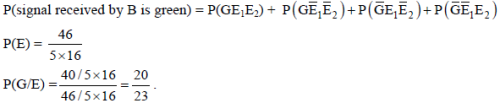QUESTION: 41

Two adjacent sides of a parallelogram ABCD are given by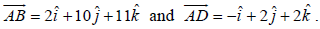The side AD is rotated by an acute angle α in the plane of the parallelogram so that AD becomes AD′. If AD′ makes a right angle with the side AB, then the cosine of the angle α is given by

Solution: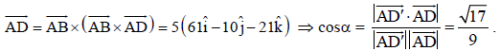QUESTION: 42

For r = 0, 1, …, 10, let Ar, Br and Cr denote, respectively, the coefficient of xr in the expansions of (1 + x)10,
(1 + x)20 and (1 + x)30.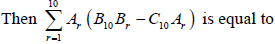is equal to

Solution: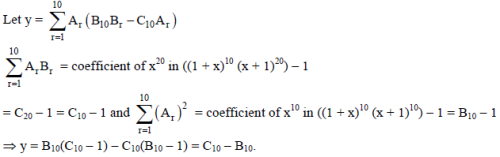QUESTION: 43

Let f be a real-valued function defined on the interval (− 1, 1) such that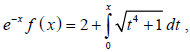for all x ∈ (−1 , 1) and let f−1 be the inverse function of f. Then (f-1)′ (2) is equal to

Solution: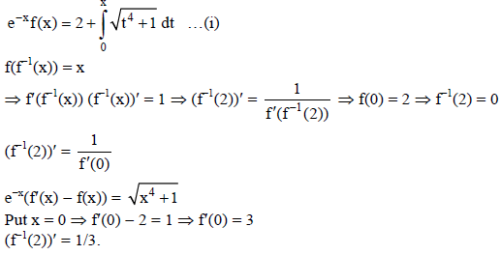QUESTION: 44

Let S = {1, 2, 3, 4}. The total number of unordered pairs of disjoint subsets of S is equal to

Solution:

Total number of unordered pairs of disjoint subsets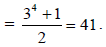*Answer can only contain numeric values
QUESTION: 45

SECTION – II (Integer Type)

This Section contains 5 questions. The answer to each question is a single-digit integer, ranging from 0 to 9.

Enter only the numerical value in the space provided below.

Q. Let a1, a2, a3, …, a11 be real numbers satisfying a1 = 15, 27 − 2a2 > 0 and ak = 2ak−1 − ak−2 for k = 3, 4, …,
11.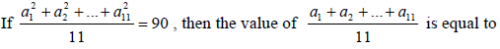Solution: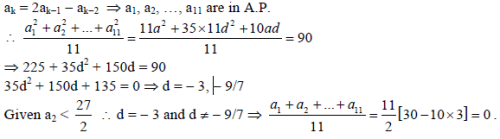*Answer can only contain numeric values
QUESTION: 46

Let f be a function defined on R (the set of all real numbers) such that f′(x) = 2010 (x − 2009) (x − 2010)2
(x − 2011)3 (x − 2012)4, for all x ∈ R. If g is a function defined on R with values in the interval (0, ∞) such
that f (x) = ln (g (x)), for all x ∈ R, then the number of points in R at which g has a local maximum is

Solution: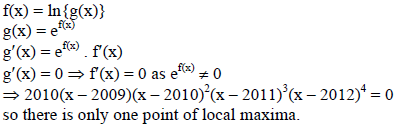*Answer can only contain numeric values
QUESTION: 47

Let k be a positive real number and let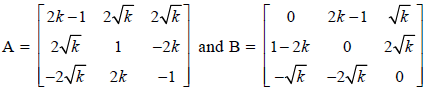. If det (adj A) + det (adj B) = 106, then [k] is equal to

[Note : adj M denotes the adjoint of a square matrix M and [k] denotes the largest integer less than or equal to k].

Solution: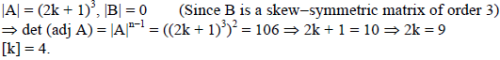*Answer can only contain numeric values
QUESTION: 48

Two parallel chords of a circle of radius 2 are at a distance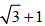apart. If the chords subtend at the centre,
angles of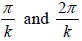, where k > 0, then the value of [k] is

[Note : [k] denotes the largest integer less than or equal to k].

Solution: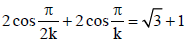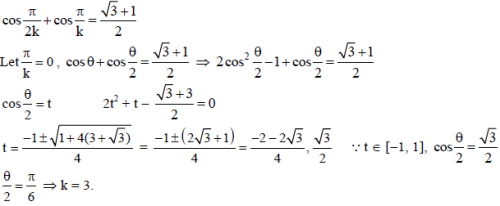*Answer can only contain numeric values
QUESTION: 49

Consider a triangle ABC and let a, b and c denote the lengths of the sides opposite to vertices A, B and C
respectively. Suppose a = 6, b = 10 and the area of the triangle is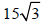. If ∠ACB is obtuse and if r denotes
the radius of the incircle of the triangle, then r2 is equal to

Solution: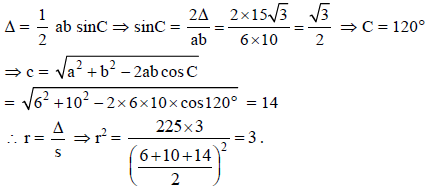QUESTION: 50

SECTION – III (Paragraph Type)

This Section contains 2 paragraphs. Based upon each of the paragraphs 3 multiple choice questions have to be answered. Each of these questions has four choices (A), (B), (C) and (D) out of which ONLY ONE is correct.

Paragraph for questions 50 to 52.

Consider the polynomial f(x) = 1 + 2x + 3x2 + 4x3. Let s be the sum of all distinct real roots of f(x) and let t = |s|.

Q. The real number s lies in the interval

Solution: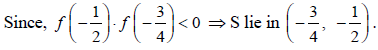QUESTION: 51

Paragraph for questions 50 to 52.

Consider the polynomial f(x) = 1 + 2x + 3x2 + 4x3. Let s be the sum of all distinct real roots of f(x) and let t = |s|.

Q. The area bounded by the curve y = f(x) and the lines x = 0, y = 0 and x = t, lies in the interval

Solution: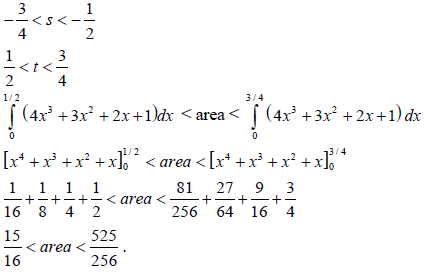QUESTION: 52

Paragraph for questions 50 to 52.

Consider the polynomial f(x) = 1 + 2x + 3x2 + 4x3. Let s be the sum of all distinct real roots of f(x) and let t = |s|.

Q. The function f′(x) is

Solution:

f″(x) = 2[12x + 3] = 0 ⇒ x = − 1/4.

QUESTION: 53

Paragraph for questions 53 to 55.

Tangents are drawn from the point P(3, 4) to the ellipse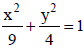touching the ellipse at points A and B.

Q. The coordinates of A and B are

Solution: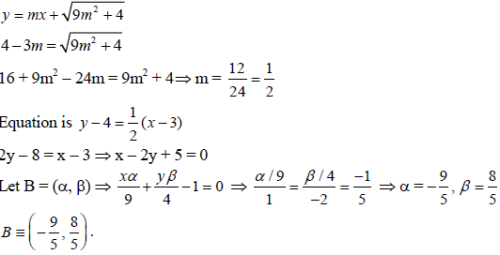QUESTION: 54

Paragraph for questions 53 to 55.

Tangents are drawn from the point P(3, 4) to the ellipsetouching the ellipse at points A and B.

Q. The orthocentre of the triangle PAB is

Solution:
QUESTION: 55

Paragraph for questions 53 to 55.

Tangents are drawn from the point P(3, 4) to the ellipsetouching the ellipse at points A and B.

Q. The equation of the locus of the point whose distances from the point P and the line AB are equal, is

Solution: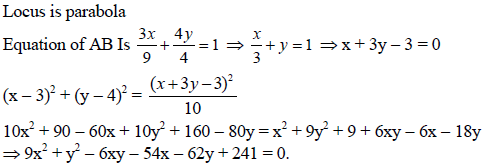QUESTION: 56

SECTION – IV (Matrix Type)

This Section contains 2 questions. Each question has four statements (A, B, C and D) given in Column I and five statements (p, q, r, s and t) in Column II. Any given statement in Column I can have correct matching with one or more statement(s) given in Column II.

Q. Match the statements in column-I with those in column-II.
[Note: Here z takes the values in the complex plane and Im z and Re z denote, respectively, the imaginary
part and the real part of z]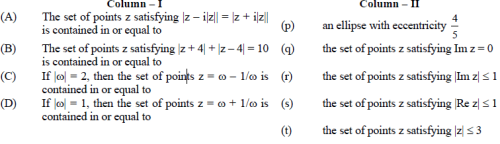Solution: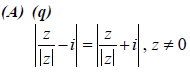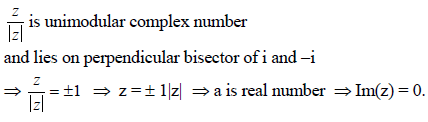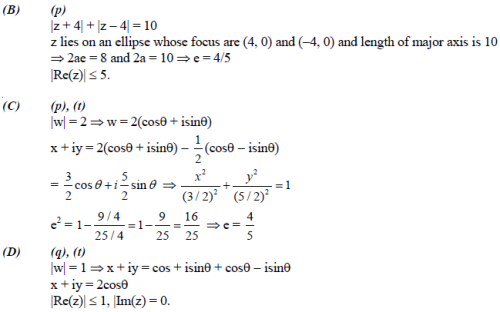QUESTION: 57

Match the statements in column-I with those in column-II.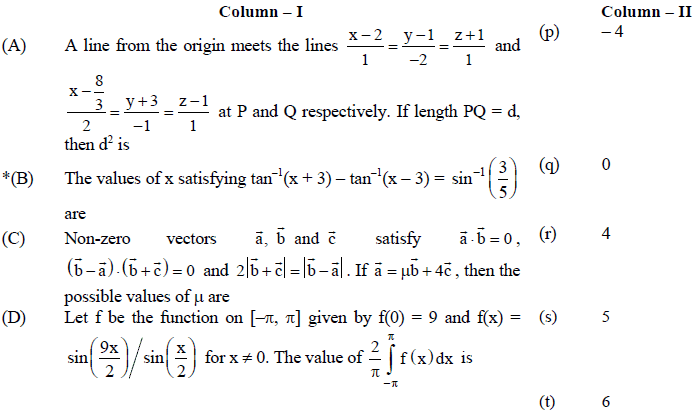Solution: